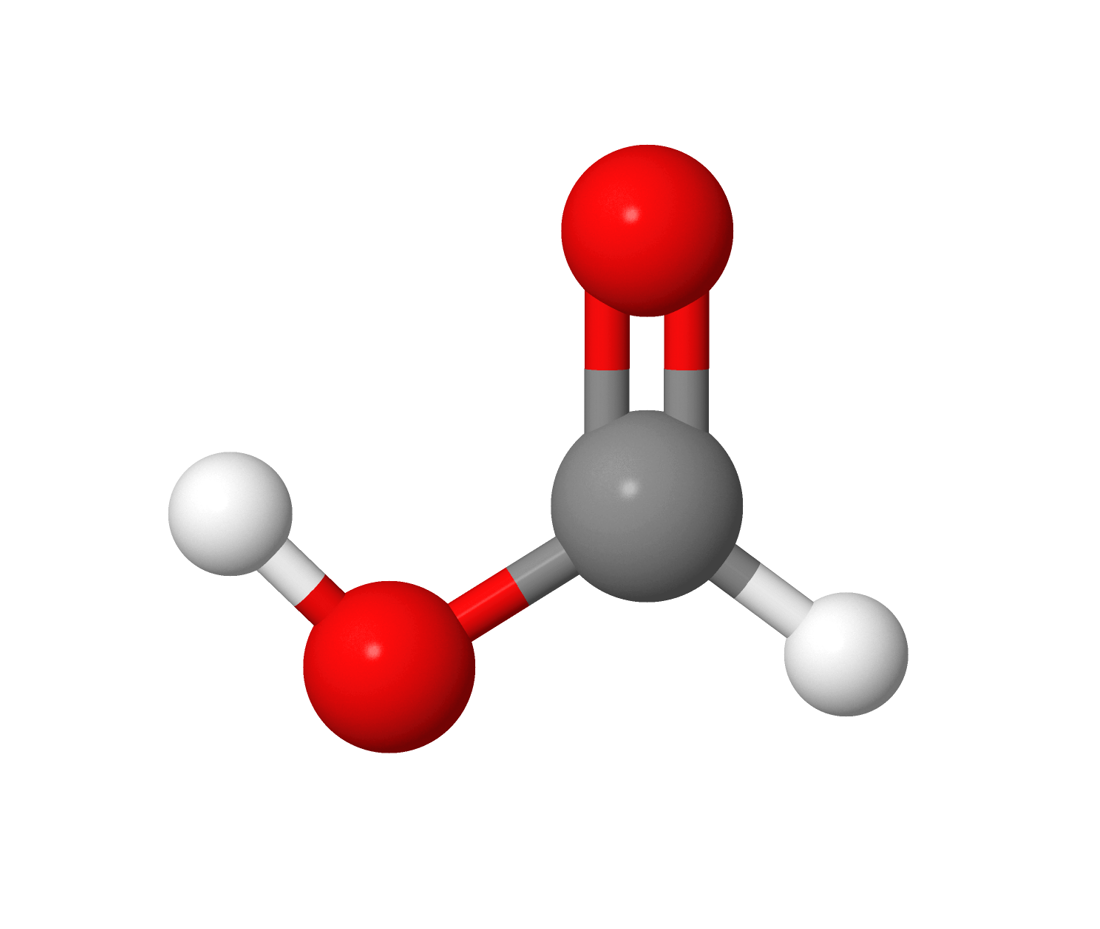# sf.apps.data.Formic¶

class Formic[source]

Zero temperature formic acid.

The molecular parameters are obtained from Ref. .

Molecule:n_mean = 1.56
threshold = False
n_samples = 20000
modes = 14
T = 0
T = 0
n_mean = 1.56
threshold = False
 counts([axis]) Count number of photons or clicks.
counts(axis=1)

Count number of photons or clicks.

Counts number of photons/clicks in each sample (axis==1) or number of photons/clicks in each mode compounded over all samples (axis==0).

Parameters

axis (int) – axis to perform count

Returns

counts from samples

Return type

list